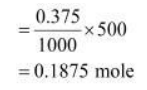# Calculate the mass of sodium acetate $\left(\mathrm{CH}_{3} \mathrm{COONa}\right)$ required to make

Question.

Calculate the mass of sodium acetate $\left(\mathrm{CH}_{3} \mathrm{COONa}\right)$ required to make $500 \mathrm{~mL}$ of $0.375$

molar aqueous solution. Molar mass of sodium acetate is $82.0245 \mathrm{~g} \mathrm{~mol}^{-1}$

Solution:

0.375 M aqueous solution of sodium acetate

≡ 1000 mL of solution containing 0.375 moles of sodium acetate

Number of moles of sodium acetate in 500 mLMolar mass of sodium acetate $=82.0245 \mathrm{~g}$ mole $^{-1}$ (Given)

$\therefore$ Required mass of sodium acetate $=\left(82.0245 \mathrm{~g} \mathrm{~mol}^{-1}\right)(0.1875 \mathrm{~mole})$

$=15.38 \mathrm{~g}$